# Mixed fractions calculator

The calculator performs basic and advanced operations with mixed numbers, fractions, integers, decimals. Mixed fractions are also called mixed numbers. A mixed fraction is a whole number and a proper fraction combined, i.e. one and three-quarters. The calculator evaluates the expression or solves the equation with step-by-step calculation progress information. Solve problems with two or more mixed numbers fractions in one expression.

## Result:

### 1/4 + 1/6 = 5/12 ≅ 0.4166667

Spelled result in words is five twelfths.

### Calculation steps

1. Add: 1/4 + 1/6 = 1 · 3/4 · 3 + 1 · 2/6 · 2 = 3/12 + 2/12 = 3 + 2/12 = 5/12
For adding, subtracting, and comparing fractions, it is suitable to adjust both fractions to a common (equal, identical) denominator. The common denominator you can calculate as the least common multiple of both denominators - LCM(4, 6) = 12. In practice, it is enough to find the common denominator (not necessarily the lowest) by multiplying the denominators: 4 × 6 = 24. In the next intermediate step, the fraction result cannot be further simplified by canceling.
In words - one quarter plus one sixth = five twelfths.

#### Examples:

sum of two mixed numbers: 1 3/4 + 2 3/8
addition of three mixed numbers: 1 3/8 + 6 11/13 + 5 7/8
addition of two mixed numbers: 2 1/2 + 4 2/3
subtracting two mixed numbers: 7 1/2 - 5 3/4
multiplication of mixed numbers: 3 3/4 * 2 2/5
comparing mixed numbers: 3 1/4 2 1/3
changing improper fraction to mixed number: 9/4
What is 3/4 as a mixed number: 3/4
subtracting mixed number and fraction: 1 3/5 - 5/6
sum mixed number and an improper fraction: 1 3/5 + 11/5

## Mixed fractions in word problems:Add this two mixed numbers: 1 5/6 + 2 2/11=
• A more than bWhat is 5 1/7 more than 2 3/7?
• A science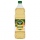A science experiment calls for making 3 and 2/3 cups of distilled water with 1 and 3/5 cups of vinegar and 2/3 cups of liquid detergent how much liquid in all and cups is needed?Add this two mixed numbers: 1 5/6 + 2 2/11=Add two mixed fractions: 2 4/6 + 1 3/62 and 1/8th plus 1 and 1/3rd =
• Jack planted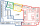Jack planted 1 1/4 rows of lettuce in the family garden, Rose planted 1 1/2 rows of broccoli and Calvin planted 4 5/12 rows of beans. How many rows were planted in the garden?
• Sum of mixedThe sum of two mixed numbers is 15½. One of the number is 5 ¾, what is the other?
• A full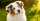A full bag of dog food weights 5 1/2 pounds. How much dog food do you have if there are 3 3/4 bags in the cupboard?3 3/4 + 2 3/5 + 5 1/2 Show your solution.
• Team runThe first team member in a 926-person relay race must run 2 1/4 laps, the second team member must run 1 1/2 laps, and the third team member must run 3 1/4 laps. How many laps in all must each team run?
• Donuts 3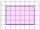You pick up donuts for a party. You have 3 and 4/12 boxes of donuts. Roy picks up donuts too. He has 2 and 9/12 boxes of donuts. How many boxes of donuts do you have in all?
• Sum of 18Sum of two fractions is 4 3/7. If one of the fractions is 2 1/5 find the other one .
• Sum of 20Sum of the two numbers is 15 1/6. If one of the numbers is 2 2/3, find the other number.
• Zailene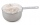Zailene wants to make two kinds of kakanin, puto and kutsina. Puto recipe needs 3 2/5 cup of flour while Kutsinta needs 5 ¼ cup of flour. How many cups of flour will Zailene be needed in all?
• Luana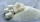Luana is making a recipe that uses 2 7/8 cups of sugar. She has 1 1/4 cups of sugar in her sugar bowl and 7 1/2 cups of sugar in the canister. How many cups of sugar will Luana have left after she makes her recipe?
• A textileA textile store sold a bolt of denim (what jeans are made out of). In one day, the following number of yards were purchased from the one bolt: 5 2/3, 7, 4 2/3, 8 5/8, 9 3/5, 10 ½, and 8. How many yards were sold?